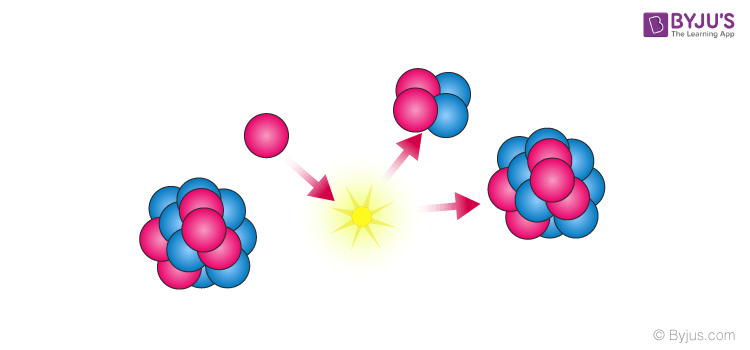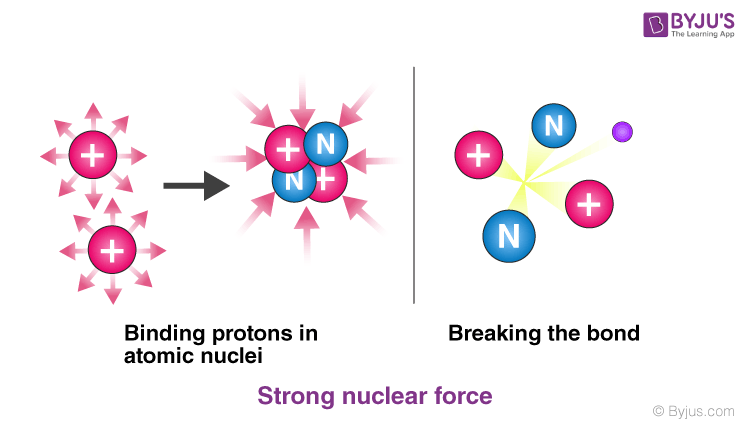# Nuclear Force

Nuclear forces (also known as nuclear interactions) are the forces that act between two or more nucleons. They bind protons and neutrons ( together called nucleons) into the atomic nuclei. The nuclear force is a short-range force and causes the stability of the nucleus. In this article, we will learn about the definition, properties and examples of nuclear forces.

## What Is Nuclear Force?

The nuclear force is one of the four fundamental forces of nature, the others being gravitational and electromagnetic forces. In fact, being 10 million times stronger than the chemical binding forces, they are also known as the strong forces. In this section, we will discuss this force in detail. We can define nuclear force as:

The nuclear force is a force that acts between the protons and neutrons of atoms.The nuclear force is the force that binds the protons and neutrons in a nucleus together. This force can exist between protons and protons, neutrons and protons or neutrons and neutrons. This force is what holds the nucleus together.

The charge of protons, which is +1e, tends to push them away from each other with a strong electric field repulsive force, following Coulomb’s law. But nuclear force is strong enough to keep them together and to overcome that resistance at short range.## Properties of Nuclear Force

• It is attractive in nature but with a repulsive core. That is the reason that the nucleus is held together without collapsing in itself.
• The range of a nuclear force is very short. At 1 Fermi, the distance between particles in a nucleus is tiny. At this range, the nuclear force is much stronger than the repulsive Coulomb’s force that pushes the particles away. However, if the distance is anything more than 2.5 Fermi, nuclear force is practically non-existent.
• The nuclear force is identical for all nucleons. It does not matter if it is a neutron or proton, once the Coulomb resistance is taken into consideration, nuclear force affects everything in the same way.
• At a distance of less than 0.7 Fermi, this force becomes repulsive. It is one of the most interesting properties of nuclear force, as this repulsive component of the force is what decides the size of the nucleus. The nucleons come closer to each other to the point that the force allows, after which they cannot come any closer because of the repulsive property of the force.Strong Nuclear force

## Nuclear Force Examples

The most obvious example of Nuclear Force, as discussed earlier, is the binding of protons, which are repulsive in nature because of their positive charge.

• On a larger scale, this force is responsible for the immense destructive power of nuclear weapons. The release of energy when a nuclear weapon is detonated is due to strong nuclear forces. It is also used in Nuclear power plants to generate heat for the purpose of generating energy, such as electricity.
• A weaker nuclear force can transform a neutron into a proton and a proton into a neutron. These forces occur in many reactions such as radioactive decay, burning of the sun, radiocarbon dating etc.
Test your Knowledge on Nuclear Force